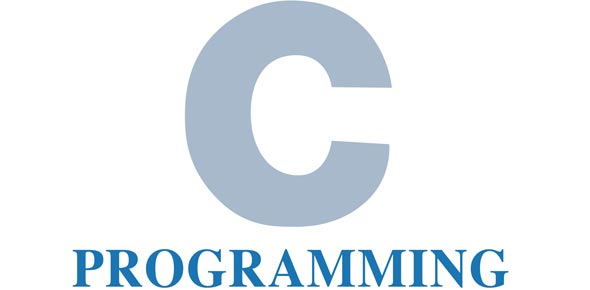# C Programming Language Challenge Quiz

10 Questions | Attempts: 206SettingsDid you know that the C programming language is a general procedural computer programming language supporting structured programming? It has discovered maximum use in applications that were formerly encoded in assembly language. This application includes operating systems and different application software for computer experts. C is an imperative procedural language. It was created to be recorded to deliver low-level access to memory and language. Put your knowledge to the test and take this quiz on C programming language.

• 1.
main() {             char *ptr1 = “abcdef”;             ptr1 = ptr1 + (strlen(ptr1)-1); printf(“%c”,--*ptr1--); printf(“%c”,--*--ptr1); printf(“%c”,--*(ptr1--)); printf(“%c”,--*(--ptr1)); printf(“%c”,*ptr1);      }
• A.

Fedcb

• B.

Ecbba

• C.

Ecbaa

• D.

None of these

• 2.
main () {             int a;             a = 3;             e(a); }   void e(int a) {             if(n>0) {                         e(--n);                                                                printf(“%d”,n);                         e(--n);             } }
• A.

0123

• B.

0120

• C.

Error

• D.

None of these

• 3.
main() {             char a[]=”abcdef”;             char * p = a;             p++;                                                     p++; p = ‘z’; printf(“ %s “,p); }
• A.

Abzdef

• B.

Zdef

• C.

Cdzf

• D.

Error

• 4.
void t1 (char *); main() {             char * p;             p = ”abcdef”;             t1(p); }   void t1(char *q) {             if(*q != ‘d’) {                         putchar(*q);                         t1(q++);             } }
• A.

Abc

• B.

Aaa

• C.

Infinite a

• D.

Error

• 5.
main () {             printf (“%d”, sizeof (65555));             printf(“%d”,sizeof(65555L));             printf(“%d”,sizeof(6.5F));             printf (“%d”,sizeof (6.5));             printf(“%d”,sizeof(6.5L)); }
• A.

2 4 4 4 8

• B.

2 4 4 4 10

• C.

4 4 4 8 10

• D.

None of these

• 6.
main() {             int q, i, j, count;             i=j=0;             q=2;             count = 6;             switch( 3 )             {                         case 0:                                     while ( --count > 0 ) {                                                 case 1:                                                             ++j;                                                 case 2:                                                             ++i;                                                 case 3:  ;                                                 case 4:  ;                                                 case 5:  ;                                     }             }                         printf(“%d”,i);             printf(“%d”,j); }
• A.

66

• B.

55

• C.

00

• D.

None of These

• 7.
Which of the following does not have an unary operator?
• A.

-7

• B.

++j

• C.

J

• D.

!i

• 8.
A union consists of a number of elements that
• A.

All occupy the same space in memory

• B.

Must be structure

• C.

Are grouped next to each other in memory

• D.

All have the same type

• 9.
The int type of constraints are whole numbers in the range
• A.

-23677 to 23678

• B.

-32768 to 32767

• C.

-32767 to 32768

• D.

-32864 to 32864

• 10.
If the variables i,j and k are assigned the values 5,3 and 2 respectively, then the expression i=j+(k++ =6)+7;
• A.

Assigns a value 16 to i

• B.

Assigns a value 18 to i

• C.

Assigns a value 19 to i

• D.

Gives an error message

## Related TopicsBack to top
×

Wait!
Here's an interesting quiz for you.